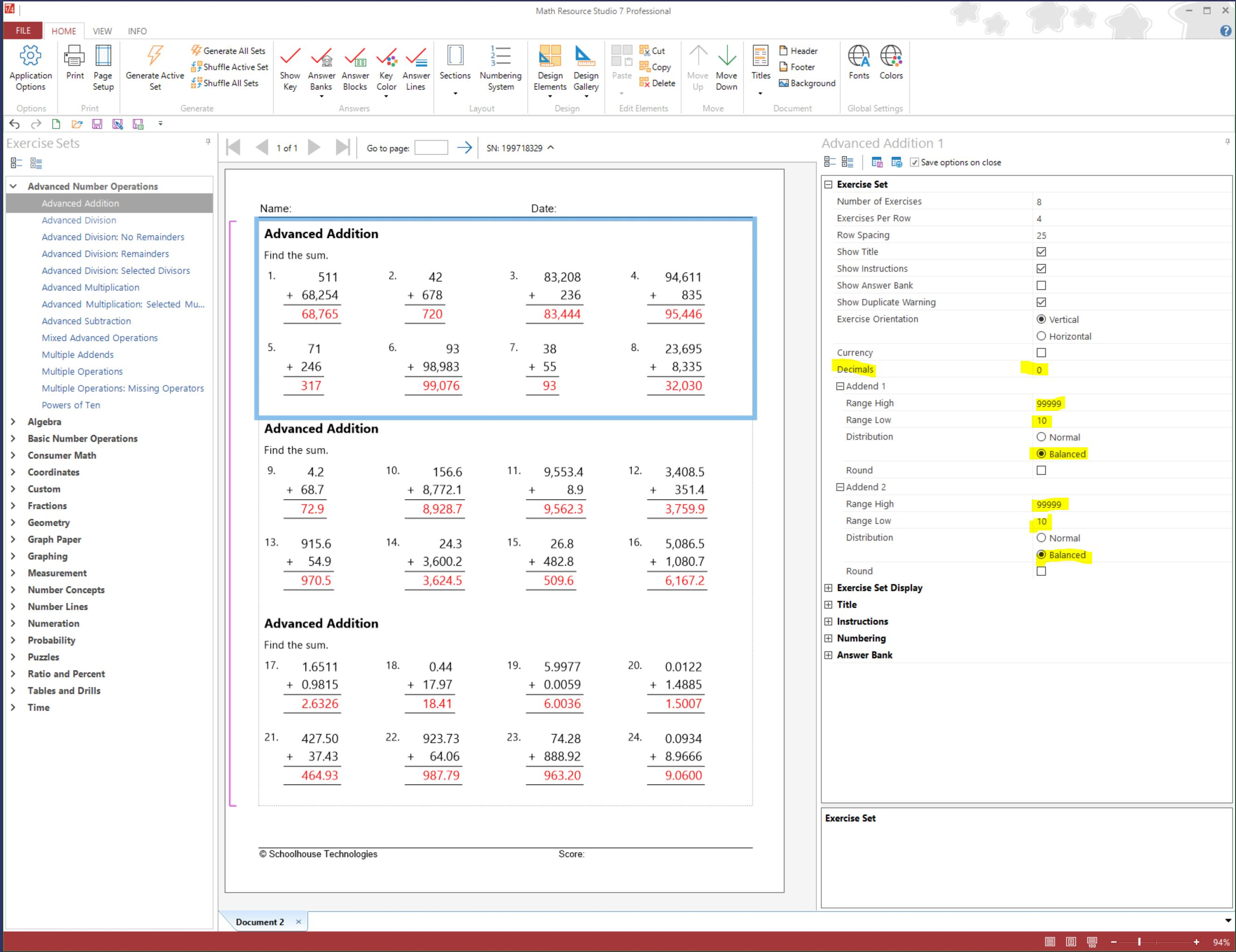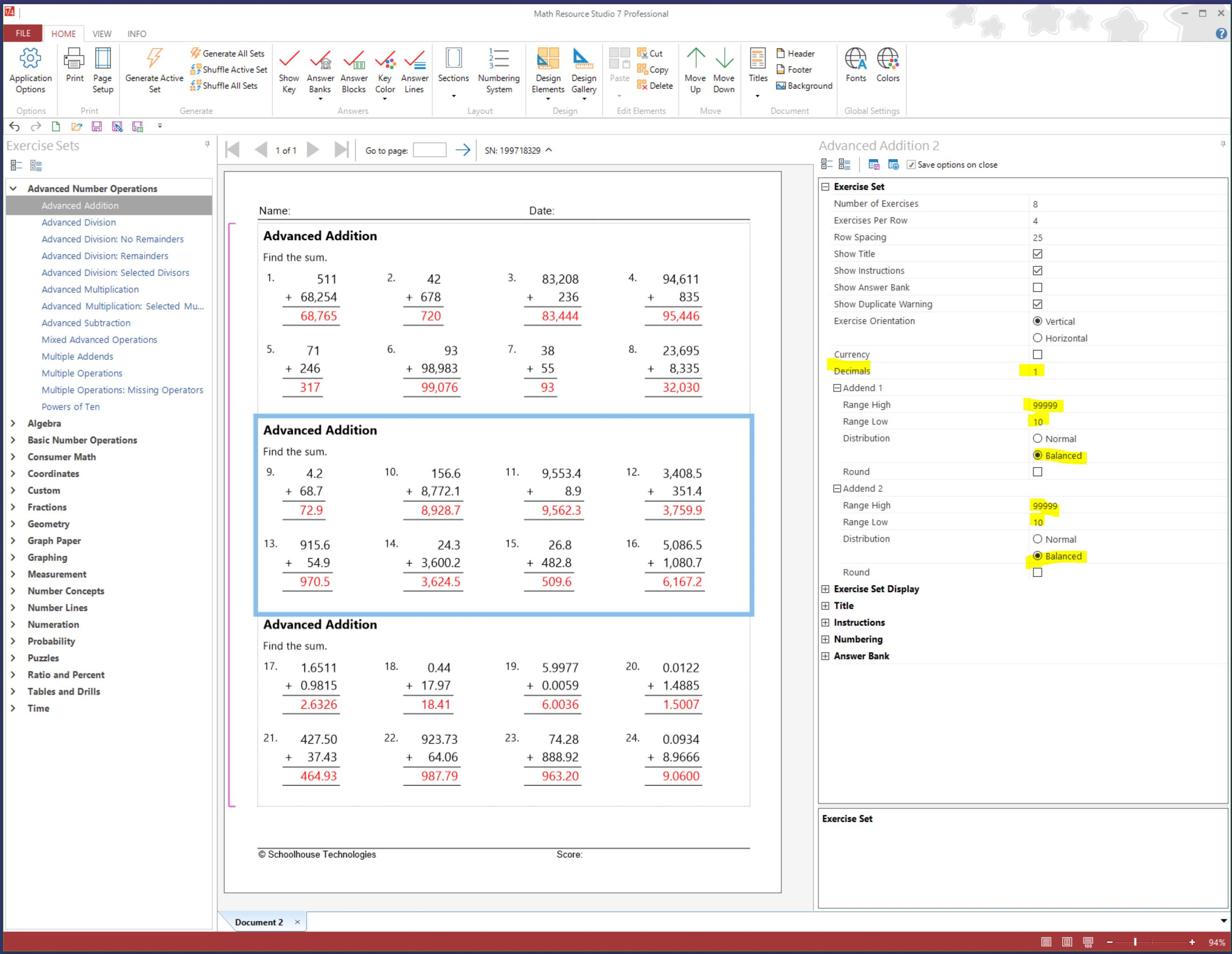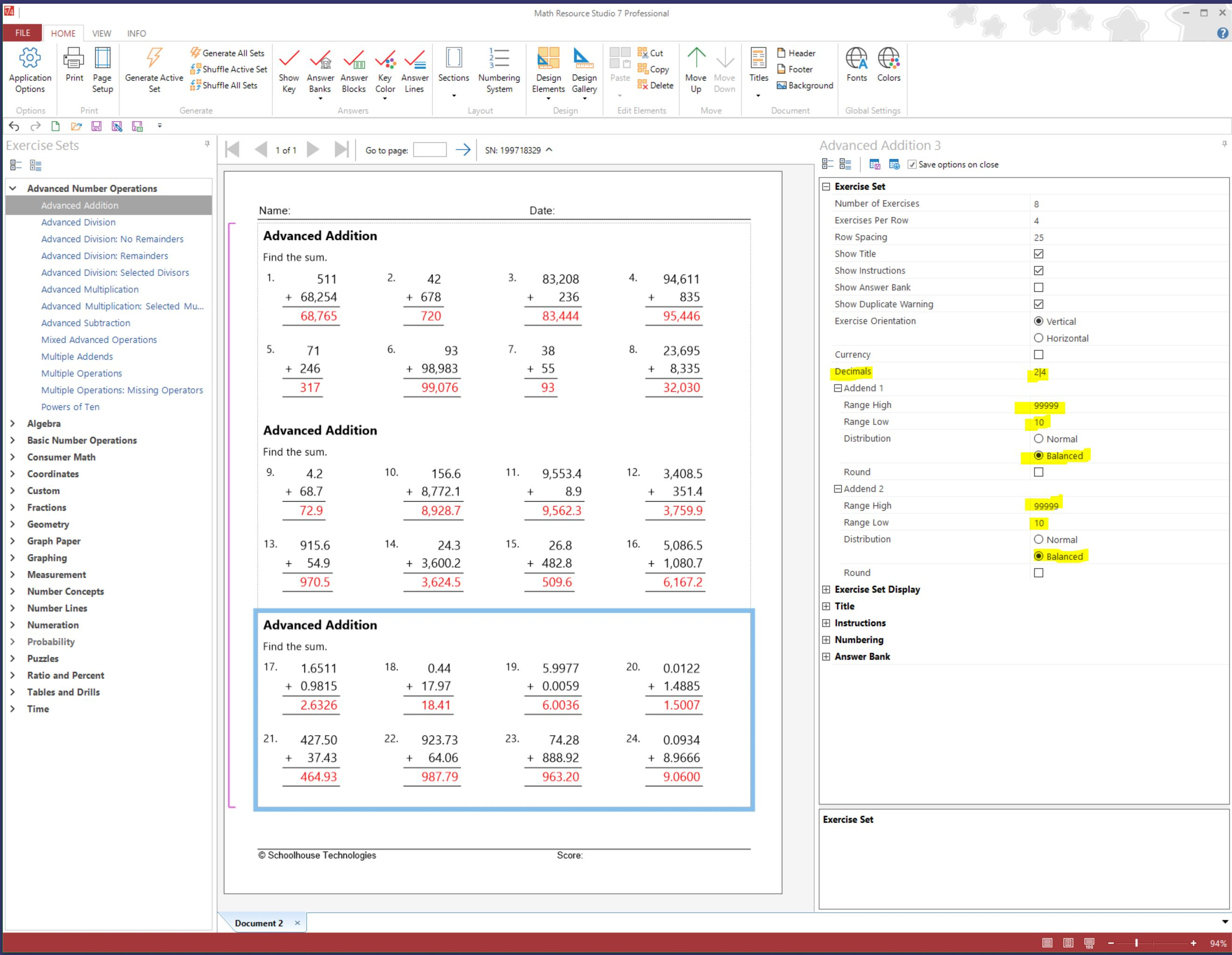# Advanced Number Operations and Decimals

If your students need practice working with decimals in equations, you will want to work with Advanced Number Operations.

Below are three pictures that show Advanced Addition with the same settings for Addend 1 and Addend 2, but the number of Decimals vary - no decimals, one decimal, and then 2 & 4 decimals. The program first determines how many decimals are requested, then generates numbers based on the number of Decimals and then the ranges for Addend 1 and Addend.

Example - If you have a number like 256:

• select 1 decimal place, the number will become 25.6
• select 2 decimal places and the number will become 2.56
• select 4 decimal places and the number will become 0.0256

Example -  All generated numbers should be similar to 759.56 and 297.36: set Decimals to two; both Addend 1 and Addend 2 ranges must be set to Range Low 10000 and Range High 99999. When using a large range of values, we recommend setting Distribution to Balanced.

Go ahead and experiment with the Decimal and Range settings to have Math Resource Studio generate the variety of numbers you want for your exercise set.